Just as all particles do not interact through electromagnetic force, all particles do not interact through the strong force either. Therefore, it is possible to classify particles into two categories - particles that can interact via the strong force and other particles that cannot. The particles that can interact with the strong force are called hadrons and other particles that cannot feel the strong force are called leptons.

### Subsection58.3.1Leptons

Electron is the prime example of a lepton. Until the discovery of muons in 1936 by Carl D. Anderson and Seth Neddermeyer at Caltech, electron and its antiparticle, positron were the only known leptons. Muon was like a heavier electron, about 200 times heavier than the electron with the same spin and same charge. The name lepton was suggested for this group of particles to indicate they were less massive than other fundamental particles'' at the time, viz., proton and neutron. But, with the discovery of the tau ($\tau$) lepton, this is no longer true.

Currently, we know of six leptons and their antiparticles in nature. Known leptons are listed in Table 58.3.1 along with some important properties. Note that for every lepton, there is a corresponding anti-lepton as well with the opposite electrical charge. All leptons are spin 1/2 particles and are generally lighter (i.e. less mass) than the hadrons. No internal structure of leptons have been found. Thus, we can think of leptons as truly elementary and point-like. Electron and the neutrinos are stable particles while muon and tau leptons decay by weak interaction.

The six leptons are referred to as six flavors. They fall into three groups, called families. In each family, there is a charged member and a neutral member. For instance, the first family has an electron and a neutrino, called electron-neutrino. In the second family is the muon and its associated mu-neutrino, and in the third family, we have the tau lepton and its associated tau-neutrino.

\begin{equation} \text{Lepton families:}\ \ \left( \begin{array}{l} e^{-}\\ \nu_e \end{array} \right),\ \ \left( \begin{array}{l} \mu^{-}\\ \nu_{\mu} \end{array} \right),\ \ \left( \begin{array}{l} \tau^{-}\\ \nu_{\tau} \end{array} \right)\tag{58.3.1} \end{equation}

#### Subsubsection58.3.1.1Lepton Family Number Conservation

Suppose we assign the two members of the electron family, viz., $e^{-}$ and $\nu_e\text{,}$ an electron-lepton number, $L_e = +1$ and to their antiparticles, viz., $e^{+}$ and $\bar\nu_e\text{,}$ the electron-lepton number, $L_e = -1\text{.}$

\begin{gather*} e^{-},\ \nu_e:\ \ L_e = + 1\\ e^{+},\ \bar\nu_e:\ \ L_e = - 1 \end{gather*}

Then, we find that, in any decay process or a nuclear reaction in which electron or electron-neutrino or their antiparticles are involved, the value of $L_e$ before the process is always equal to the value after the process. This is the conservation of electron-lepton number. We have similar conservation laws for the other two families, namely, the muon-lepton number $L_{\mu}$ and the tau-lepton number $L_{\tau}\text{.}$

\begin{align*} \amp \mu,\ \nu_\mu:\ \ L_\mu = + 1\\ \amp \bar\mu,\ \bar{\nu}_\mu:\ \ L_\mu = - 1\\ \amp \tau,\ \nu_\tau:\ \ L_\tau = + 1\\ \amp \bar\tau,\ \bar{\nu}_\tau:\ \ L_\tau = - 1 \end{align*}

Here are some examples of lepton reactions which illustrate lepton number conservation.

\begin{align*} \amp \text{Ex 1. Muon decay: } \mu^{-1} \longrightarrow e^{-1} + \bar\nu_e + \nu_{\mu}\\ \amp \text{Left: } L_e=0,\ L_\mu=1,\ \ \text{Right: } L_e=1 +(-1)=0,\ L_\mu = -1. \\ \amp \text{Ex 2. Electron capture: } \text{p} + e^{-1} \longrightarrow \text{n} + \nu_e\\ \amp \text{Left: } L_e = 0 + 1 = 1,\ \ \text{Right: } L_e = 0 + 1 = 1. \end{align*}

Hadrons are the particles that do interact through the strong force. They may also interact via weak and electromagnetic forces. We further subdivide hadrons based on whether the hadron is a fermion, meaning spin be an odd multiple of $\frac{1}{2}\text{,}$ or a boson, meaning spin be an integer, including zero. The hadrons that are fermions are called baryons and the bosonic hadrons are called mesons.

Actually, all baryons have spin either $\frac{1}{2}$ or $\frac{3}{2}$ and all mesons have spin either 0 or 1. The mesons are also lighter than baryons. Proton and neutron are familiar examples of baryons, pions and kaons are examples of mesons. Table 58.3.2 contains several more examples of baryons and mesons with their masses and other properties. Antibaryons and antimesons have opposite charge, baryon number and strangeness. Proton is the only stable hadron. All mesons decay into leptons through the weak interaction. All baryons, except protons, decay so that one of the products is proton. The study of decays and scattering reveals additional conservation laws.

#### Subsubsection58.3.2.1Baryon Number Conservation

Suppose we count a baryon as +1 and an antibaryon as -1. We call these values baryon number $(B)$ of the particle. \index{Baryon number} Then, we find that, in every decay or nuclear reaction in which hadrons participate, the number of baryons before the process is equal to the number of baryon after the process. That is, baryon number is conserved. Here are some examples:

\begin{equation*} \begin{array}{lccc} \text{Beta decay: } \amp \text{n} \amp \longrightarrow \amp \text{p} + e^{-1} + \bar\nu_e\\ \amp B = +1 \amp \amp B = +1\ \ \ \ \ \ \ \ \\ \text{Electron capture: } \amp \text{p} + e^{-1} \amp \longrightarrow \amp \text{n} + \nu_e\\ \amp B = +1 \amp \amp B = +1\ \ \ \ \ \ \ \ \end{array} \end{equation*}

#### Subsubsection58.3.2.2Strange Conservation

Early hunting for elementary particles was done in the particles produced when cosmic rays struck particles in the atmosphere. For instance, this is how the positron was discovered. In these studies it was also noted that all baryons, except proton, decayed with a lifetime that was characteristic of the force involved in the decay process. Particles that decay by weak force have a life-time of $10^{-10}$ sec to $10^{-8}$ sec and could be seen on tracks made in the bubble chamber. On the other hand, if a particle decays by strong force, the life-times of such particles were very short, $\lt 10^{-20}$ sec; that is, these particles would be formed and decay within a time $\lt 10^{-20}$ sec. With this short a life-time, the particle would travel $\lt 10^{-12}$ m before it would disappear, and hence their tracks were not visible. You saw only the tacks of particles coming out of a point where some other particle seem to just vanish. The existence of these extremely short-lived particles were inferred only after by analyzing the decay products.

Most of the particles had a common feature that if they were generated by strong interaction, they decayed by strong interaction. However, three particles, the meson kaon (K), and the baryons lambda ($\Lambda$), and sigma $(\Sigma)\text{,}$ did not fit this pattern. Although, they formed in reactions that suggested that they formed by strong interaction, they decayed with much longer life-time, which was characteristics of the weak interaction decay.

Somehow, the decays by the strong interaction channels for K, $\Lambda\text{,}$ and $\Sigma$ were blocked or forbidden. Because of this strange behavior, these particles are called strange particles. From quantum mechanics of atoms and molecules it was known that if a quantum transition was forbidden, it takes longer if it occurs at all. For instance, the triplet to singlet transition in a molecule is forbidden, which explains the long-life of phosphorescence. A similar physics must be happening with these strange particles - perhaps these particles had a property that could not be changed by strong interaction but could be changed by weak interaction. If that were ture, it would explain why the strong channel was blocked and weak channel was open for these particles. The proposed property is called strangeness. We introduce a law of conservation of strangeness, which is obeyed by only strong and electromagnetic interactions and violated by weak interaction.

Strong interaction and electromagnetic interactions conserve strangeness but weak interaction does not.

By examining the formation and decay of the strange particles, following values were assigned to the strangeness quantum number for the three strange particles metioned above.

Particle $S$
K $+1$
$\Lambda$ $-1$
$\Sigma$ $-2$

The strangeness of some particles are given in Table 58.3.2. Here are some examples of conservation or non-conservation of strangeness.

\begin{equation*} \begin{array}{lccc} \text{Strong interaction: } \amp \pi^{-1} + p \amp \longrightarrow \amp \text{K}^{0} + \Lambda^{0} \\ \amp S = 0 + 0 \amp \amp S = +1 - 1 = 0.\ \ \ \ \ \ \ \ \\ \text{Weak interaction: } \amp K^{0} \amp \longrightarrow \amp \pi^{+} + \mu^{-} + \bar\nu_{\mu} \\ \amp S = +1 \amp \amp S = 0+0+0 = 0\ \ \ \ \ \ \ \ \\ \text{Weak interaction: } \amp \Lambda^{0} \amp \longrightarrow \amp \pi^{-} + p \\ \amp S = -1 \amp \amp S = 0+0 = 0\ \ \ \ \ \ \ \ \end{array} \end{equation*}

### Subsection58.3.3Quarks

#### Subsubsection58.3.3.1Eight-fold Way

As Table 58.3.2 shows there are many baryons and mesons. In the 1950s and 60s the list of these particles was already very long and growing every year. In 1961, Murray Gell-Mann of the United States and Yuval N\'eeman of Israel noticed symmetries in hadrons. The symmetry, now known as flavor symmetry, can be seen in Figure 58.3.3-Figure 58.3.5 when particles of a particular spin value were placed on a charge-strangeness diagram. Mesons of spin 0 and baryons of spin $\frac{1}{2}$ form a hexagon pattern with two particles at the center. The antiparticles of the particles at the center are the particles themselves, and the particles and antiparticles of other articles are at the mirror points of vertices. This pattern of octets was named Eight-fold way by Gell-Mann in allusion to the noble Eight-fold Path of Budhism.

The Eight-fold Way comes from representation of the symmetry group SU(3). When Gell-Mann and N\'eeman independently applied SU(3) group to spin $\frac{3}{2}$ particles, they noticed that the representation should have ten particles, but only nine had been discovered so far. Using the pattern, Gell-Mann and N\'eeman predicted that the missing particle would have a mass of 1680 MeV, a spin of $\frac{3}{2}\text{,}$ a charge of $-1e\text{,}$ and a strangeness of $-3\text{.}$ This gave guidance to experimentalists who quickly found the missing particle, which is the $\Omega^{-}$ baryon, the particle at the tip in Figure 58.3.5.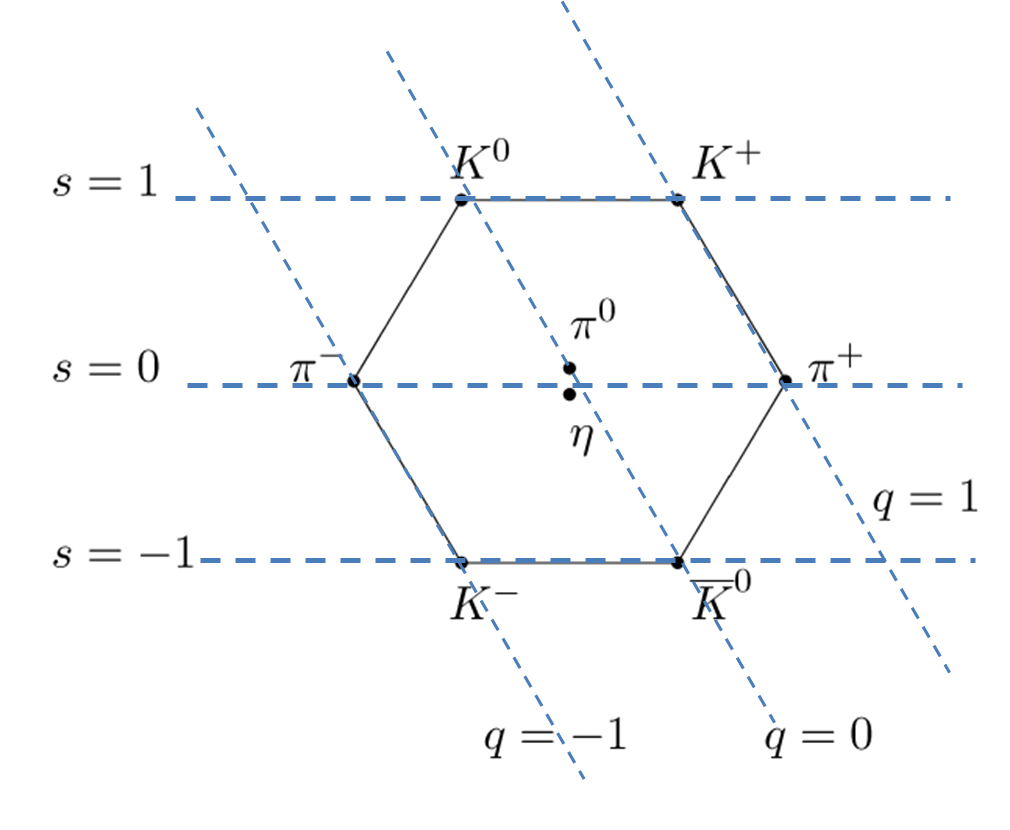Figure 58.3.3. Eight-fold way pattern for spin zero mesons.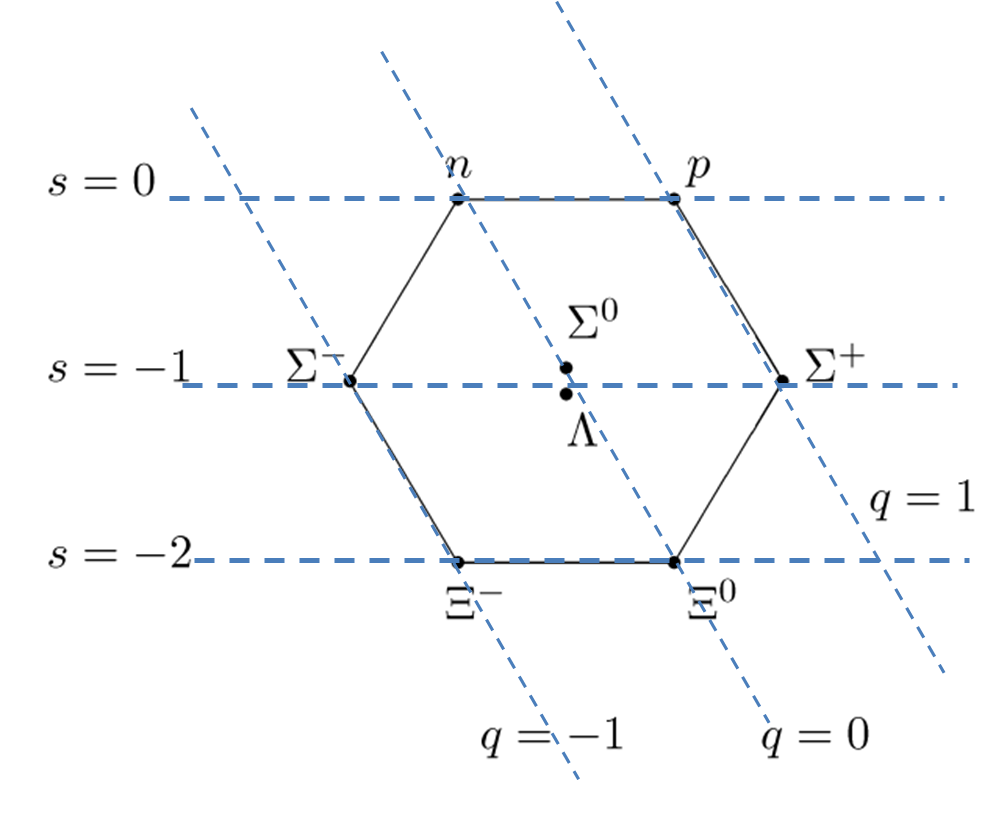Figure 58.3.4. Eight-fold way pattern for spin $\frac{1}{2}$ mesons.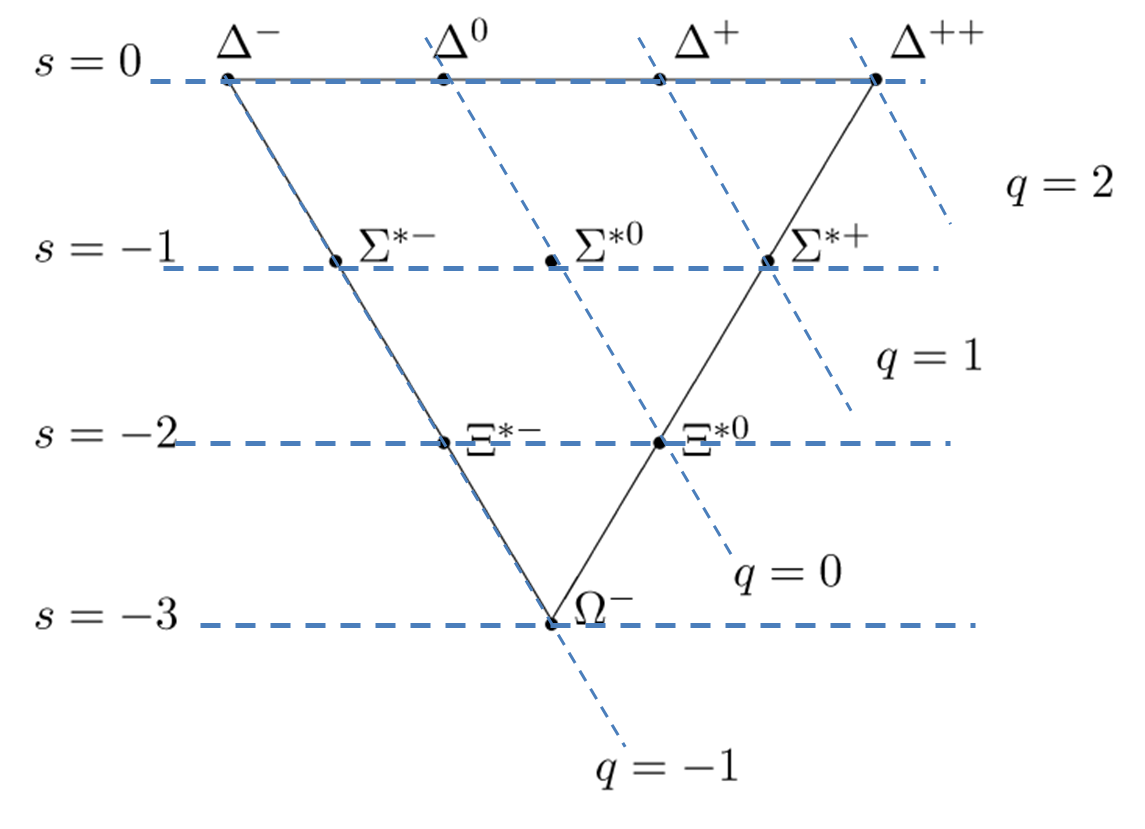Figure 58.3.5. Eight-fold way pattern for spin $\frac{3}{2}$ mesons, which is actually a 10-member system, so-called baryon decuplet.

#### Subsubsection58.3.3.2Quark flavors

In 1964 Murray Gell-Mann and George Zweig of US independently introduced the concept of physical particles inside mesons and baryons. While Gell-Mann called the constituents quarks'', Zweig called them Aces''. According to Gell-Mann, the name quark came from the passage,  Three quarks for Muster Mark...'', in James Joyce's novel Finnegan's Wake.

The models of Gell-Mann and Zweig gave a simple picture in which mesons consist of a quark and an antiquark and baryons contain three quarks as illustrated in Figure 58.3.6. In the original quark model, only three types (or flavors) of quarks were introduced, which are now called the up (u), down (d), and strange (s), to account for the mesons and baryons known at the time.

Recall the discovery of the strangeness property in the longer than expected decay time for certain particles. The particles formed in a strong interaction but decayed with weak interaction meant the decay channel by strong interaction was forbidden due to strangeness changing decay. A similar phenomenon was discovered in 1974 almost simultaneously in two separate experiments, one led by Sam Richter at Stanford Linear Accelerator (SLAC) and the other led by Sam Ting at Brookhaven National Laboratory. They found a meson of rest mass $3.0969\: \text{GeV/c}^2\text{,}$ formed by strong interaction, but decaying by weak interaction since the life time of the particle was $\sim 10^{-20}\:\text{sec}\text{,}$ which was three orders of magnitude longer than $\sim 10^{-23}\:\text{sec}$ expected if the decay occurred through strong interaction. The particle has two separate names put together in one symbol, $J/\Psi\text{.}$ A meson of this property had been predicted on theoretical grounds by Sheldon Glashow, John Iliopoulos, and Luciano Maiani in 1970 in which they had hypothesized the existence of a fourth flavor of quark, charm. The $J/\Psi$ particle is a meson which is a bound state of charm and anticharm quarks.

Just as the strange quark has a non-zero strangeness quantum number, the charm quark was assigned a new quantum property of charmness to explain its larger lifetime. The charm quark has a charmness of $+1$ and the anticharm has charmness of $-1\text{.}$ The longer lifetime of the $J/\Psi$ decay has to do with the fact that strong interaction conserves charm just as it conserved strangeness. Therefore, $J/\Psi$ cannot decay by strong interaction, and therefore it takes longer to decay and decays by weak interaction.

In 1977 a team led by Leon Lederman at Fermi National Accelerator Laboratory (also called Fermilab) discovered another meson $\Upsilon$ (upsilon) of rest mass, $9.5\: \text{MeV/c}^2\text{,}$ about ten times the rest mass of a proton, which decayed into an electron an positron pair with a lifetime $\sim 10^{-20}\:\text{sec}\text{,}$ which is not a decay by the strong interaction, by which $\Upsilon$ was formed. $\Upsilon$ is a bound state of quark bottom (b) and its antiquark, the antibottom ($\bar{b}$). The bottom quark has a property called bottomness. The bottomness of the bottom quark is $+1$ and that of the antibottom is $-1\text{.}$

In 1994/1995, yet another quark was discovered at Fermilab. When proton and antiproton beams collided at much higher energy than used for the $\Upsilon$ production, top-antitop pair was produced which decayed through weak interaction. The top quark has the rest mass of $173.34\: \text{GeV/c}^2\text{.}$ In order to explain Charge Parity (CP) violation in Kaon decays by Standard Model, Makoto Kobayashi and Toshihide Maskawa of Japan, had hypothesized the existence of the third generation of quarks, with top and bottom quarks as members. Unlike other quarks which are always produced in mesons, the top quarks is anticipated to be produced by itself.

Currently, there are six flavors of quarks known, which come in three families, similar to the families of leptons. The six quarks are : up (u), down (d), charm (c), strange (s), top (t), and bottom (b). The top and bottom are sometimes affectionately called truth and beauty respectively. Although, there is no up-ness or down-ness, all other quarks have quantum numbers associated with them, viz., charmness, strangeness, topness, bottomness, which are conserved in strong interaction. To keep the strange quarks strange, the strangeness quantum number for strange quarks is $-1$ rather than $+1\text{.}$ For all other quarks, their falvor-ness is $+1$ for that type of quark, and $-1$ for the antiquark, e.g. charm (c) has charmness $+1$ and anticharm ($\bar{c}$) has charmness $-1\text{.}$

\begin{equation*} \text{Quark families:}\ \ \left( \begin{array}{l} u\\ d \end{array} \right),\ \ \left( \begin{array}{l} c\\ s \end{array} \right),\ \ \left( \begin{array}{l} t\\ b \end{array} \right) \end{equation*}

Quarks are spin $\frac{1}{2}$ particles with fractional charge ($+\frac{2}{3}e$ and $-\frac{1}{3}e$) and fractional baryon number as listed in Table 58.3.7. The strange quark is given the strangeness of $-1$ while all other quarks have strangeness $0\text{.}$ Although quarks have not been observed in isolation, there is considerable experimental evidence of their existence.

Ordinary particles, such as proton and neutron contained up and down quarks while the strange particles contained the strange quark and one or more up and down quarks as shown in Table 58.3.8. For instance, proton has two up quarks and one down quark. The spins of the two up quarks must be opposite giving a net spin of $\frac{1}{2}$ for the proton. The charges of the three quarks add up to $2\times \frac{2}{3} - \frac{1}{3} = 1$ times the electronic charge, as expected. Similarly, neutron has one up quark and two down quarks as you can verify yourself. The antibaryon will have all antiquarks. For instance, anti proton will be $\bar{u}\bar{u}\bar{d}\text{,}$ and antineutron $\bar{u}\bar{d}\bar{d}\text{.}$

(a) Examine the beta decay of neutron ($\text{n} \rightarrow \text{p} + \text{e}^{-1} + \bar{\nu}_e$) in terms of quarks. (b) Draw a Feynman diagram to show the reaction and the type of fundamental force involved.

Hint

Solution

(a) Let us write the two hadrons in the beta decay in terms of their quark content to get a sens of what may be happening at the elementary level. $\text{udd} \rightarrow \text{uud} + \text{e}^{-1} + \bar{\nu}_e.$ Comparing the quark content we observe that one of the down quark in the neutron has converted to an up quark in the proton. Thus, we can write this equation as $d \rightarrow u + \text{e}^{-1} + \bar{\nu}_e.$ This reaction can happen only by weak interaction, which has three charge carriers, $\text{W}^{+}\text{,}$ $\text{W}^{-}\text{,}$ and $\text{Z}^{0}\text{.}$ In the conversion of d (charge $-$e/3) to u (charge $+$2e/3), the change in charge is $-$e. Therefore, a the particle $\text{W}^{-}$ will be emitted. $d \rightarrow u + \text{W}^{-},$ as each side of this elementary reaction will have net charge $-$e/3. The emitted $\text{W}^{-}$ then dissociates into an electron and an antineutrino.

(b) The Feynman diagram will show two vertices corresponding to the emission and dissociation of $\text{W}^{-}$ as drawn in Figure 58.3.10.

#### Subsubsection58.3.3.3Quark Colors

When quark content of various baryons and mesons were analyzed it was found that there are baryons that contain three quarks of the same type. For instance, the strange baryon $\Omega^{-}$ has three strange quarks in the same spin state, giving spin $\frac{3}{2}$ for $\Omega^{-}\text{.}$ Similarly, the baryon $\Delta^{++}$ has three up quarks in the same spin state so that spin of $\Delta^{++}$ is $\frac{3}{2}\text{.}$ These baryons would violate Pauli's exclusion principle for fermions, which states that no two identical particles can be in the same quantum state. To overcome this problem, Oscar W. Greenberg and Moo-Young Han and Yoichiro Nambu of US introduced the notion of color charge, or simply color, with three values so that each identical quark in a baryon could have different value and thus would avoid the violation of Pauli's exclusion principle.

The color quantum number quarks is given red, green, and blue, although they have nothing to do with the colors of light. The antiquarks have opposite colors for their color quantum number: anti-red, anti-green, and anti-blue, usually written without the hyphens, antired, antigreen, and antiblue. The anlaogy with the colors of light is further exploited by noting that mixing the three colored lights, red, green, blue, makes white (colorless) light. Thus, when a baryon containing three quarks have three different colors, the color quantum number of the baryon will be colorless as illustrated in Figure 58.3.11. The mesons also form so that they are also colorless; in mesons, the quark and antiquark have opposite colors. For instance, in $\pi^{+}$ meson, if the up quark is in red color state, then the anti-down quark will be in the anti-red color state.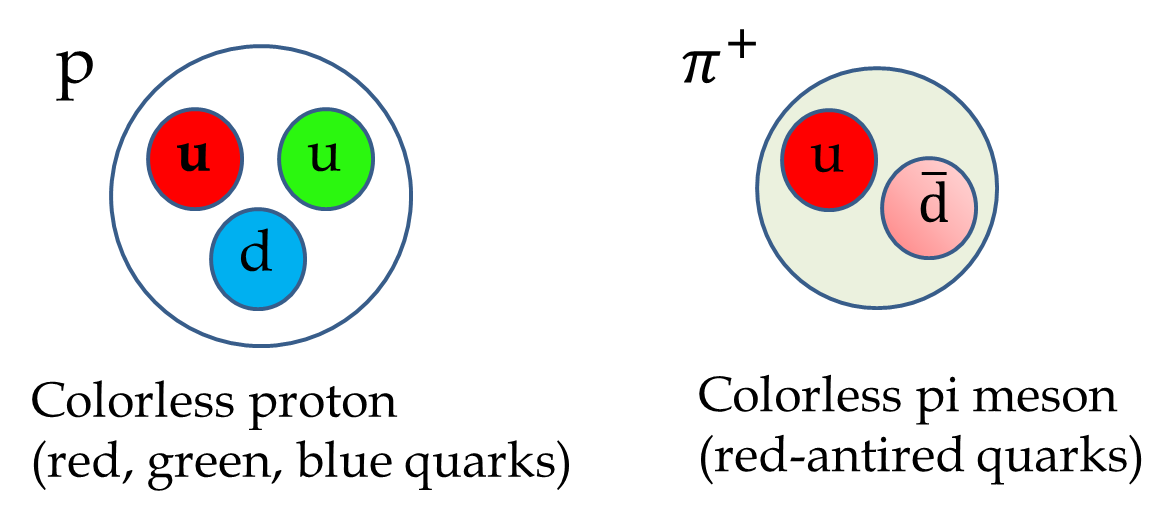Figure 58.3.11. Baryons and mesons are colorless. In baryons the three quarks have different colors and in mesons the quark and antoquark have opposite colors.

#### Subsubsection58.3.3.4Color force between quarks

Just as there is an electric force between two particles with electric charge, there is a color force between two quarks due to their color property. The color force is described by a theory of quarks called quantum chromodynamics (QCD). The color force is the fundamental way strong force acts. The force mediator particles of the color force are called gluons which are doubly colored so that they can change the color of a quark that emits or absorbs it. To exchange three colors with any other three colors it would seem we need nine gluons: red-antired, red-antigreen, red-antiblue, green-antired, green-antigree, green-antiblue, blue-antired, blue-antigree, blue-antiblue. However, the sum of three of these states, namely red-antired, green-antigreen, and blue-antiblue, is a colorless state, which means that these three states are not linearly independent and only two can be taken as independent states. This gives eight possible color-anticolor states for gluons. Quark-quark interactions are illustrated in Figure 58.3.12. At each vertex, where a quarks emits or absorbs a gluon, the net color is conserved, i.e., an algebraic sum of colors at the vertex must be zero as given in the figure.

#### Subsubsection58.3.3.5Strong force between nucleons

We have mentioned above that the strong nuclear force between nucleons is not a fundamental force but rather an effective force arizing out of the more fundamental color force between quarks. Recall the Feynman diagram in Figure 58.2.3 that showed the exchange of a pion between a proton and a neutron when they scatter off each other. Let us see how that pion exchange comes about in the quark picture. This is shown in Figure 58.3.13. First, we replace the proton and neutron by their quark content and think of a way to switch the u and d quarks without changing flavor of the quarks since the color force only changes color and not the flavor. That is, an up quark can only either remain an up quark or change to antiup quark, it cannot change to a down or any other quark. The figure shows how a proton will turn into a neutron and a neutron will turn into a proton.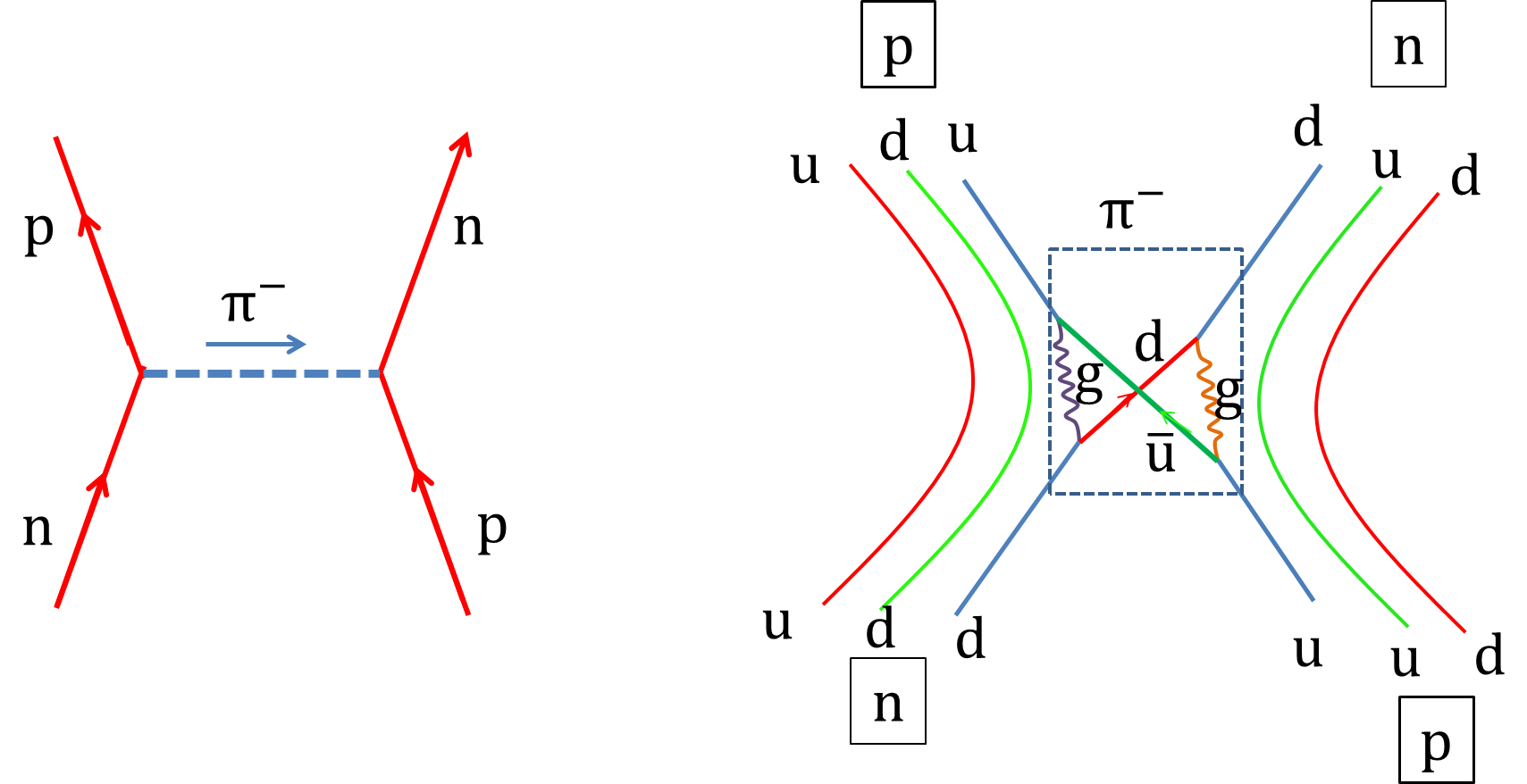Figure 58.3.13. The strong force between nucleons are fundamentally color force between quarks. The exchange of gluons and the exchange of quarks explains the quark content of the pion that must be involved for proton and neutron to interact by the strong force via $\pi^{-}$ exchange.
TODO

\​begin{exercise} Identify the conservation law(s) violated by each reaction. \​begin{enumerate}[(a)] \item $\textrm{p} + \gamma \rightarrow \textrm{n} + \textrm{e}^{-}$ \item $\bar{\textrm{p}}+ \gamma \rightarrow \textrm{n} + \textrm{e}^{+}$ \item $\mu^{-} \rightarrow \textrm{e}^{-} + \bar{\nu_e}$ \item $\tau^{-} \rightarrow \textrm{e}^{-} + \bar{\nu_e} + \bar{\nu_{\tau}}$ \end{enumerate} \end{exercise}

\​begin{exercise} Identify the conservation law(s) violated by each reaction. \​begin{enumerate}[(a)] \item $\textrm{p} + \bar{\textrm{p}} \rightarrow \textrm{n} + \textrm{e}^{+} + \textrm{e}^{-}$ \item $\Delta_0 \rightarrow \pi^{+} + \pi^{-}$ \item $\textrm{p} + \textrm{p} \rightarrow \pi^{+} + \pi^{+} + \pi^{0}$ \end{enumerate} \end{exercise}

\​begin{exercise} Identify the conservation law(s) violated by each reaction. \​begin{enumerate}[(a)] \item $\Sigma_0 \rightarrow \pi^{+} + \pi^{-}$ \item $\Omega^{-} \rightarrow \textrm{n} + \textrm{e}^{-} + \bar{\nu_e}$ \item $\textrm{p} + \textrm{p} \rightarrow \Sigma^{+} + \pi^{+}$ \item $\textrm{p} + \bar{\textrm{p} } \rightarrow \Lambda^{0} + \bar{\Lambda}^{0}$ \end{enumerate} \end{exercise}

\​begin{exercise} In the text we have discussed the production of antiproton by a beam of protons striking a proton-rich target. The reaction is normally written as $\textrm{p} + \textrm{p} \rightarrow \textrm{p} + \textrm{p} + \textrm{p} + \bar{\textrm{p}}.$ In addition to this reaction the reaction prducing antiproton also occurs. This reaction is written as $\textrm{p} + \textrm{p} \rightarrow \textrm{p} + \textrm{p} + \textrm{n} + \bar{\textrm{n}}.$ Explain why we need to include p + p on the right side of these reactions. \end{exercise}

\​begin{exercise} A negative pion at rest decays into a muon and antineutrino. (a) Write the reaction. (b) Using the quark content of the pion, show the fundamental transformations and the force particles that are involved in the reaction. (c) Draw a Feynman diagram that shows the force particles as well as the initial and final particles. (d) Calculate the energies and momenta of the muon and antineutrino. \end{exercise}

\​begin{exercise} Negative pions of kinetic energy $K_\pi$ strike a stationary proton target. If $K_\pi$ is sufficient a K and a sigma particle will be produced by the following reaction. $\pi^{-} + \textrm{p} \rightarrow \textrm{K}^0 + \Sigma^{0}.$ The rest mass energy of the pion is 139.5 MeV/c$^2$, K$^0$ is 497.7 MeV/c$^2$ and of $\Sigma^{0}$ is 1192.5 MeV/c$^2$. (a) Analyze this equation in the quark picture. (b) Draw a Feynman diagram for this reaction. (c) Use the rest masses of the reactants and products and find the value of $K_\pi$ that is required for the reaction to occur. This is the threshold energy. Use relativistic formula for kinetic energy. \end{exercise}

\​begin{exercise} A kaon at rest decays into two pions by the following reaction. $\textrm{K}^{0} \rightarrow\pi^{+} + \pi^{-} \ \ \ \ (\sim 10^{-10}\:\textrm{s}).$ The rest mass energy of K$^0$ is 497.7 MeV/c$^2$ and that pions is 139.5 MeV/c$^2$. (a) Analyze this equation in the quark picture. (b) Draw a Feynman diagram for this reaction. (c) Compute the dissociation energy and the kinetic energies of the two pions. (d) Identify type of interaction is responsible for the decay and give your reasoning. \end{exercise}

\​begin{exercise} A Lambda particle at rest decays into a proton and a pion by the following reaction. $\Lambda^{0} \rightarrow\textrm{p} + \pi^{-} \ \ \ \ (0.6\times 10^{-23}\:\textrm{s}).$ The rest mass energy of $\Lambda_0$ is 1115.7 MeV/c$^2$ and that of negative pion is 139.5 MeV/c$^2$. (a) Analyze this equation in the quark picture. (b) Draw a Feynman diagram for this reaction. (c) Compute the dissociation energy and the kinetic energies of the proton and pion. (d) Identify type of interaction is responsible for the decay and give your reasoning. \end{exercise}